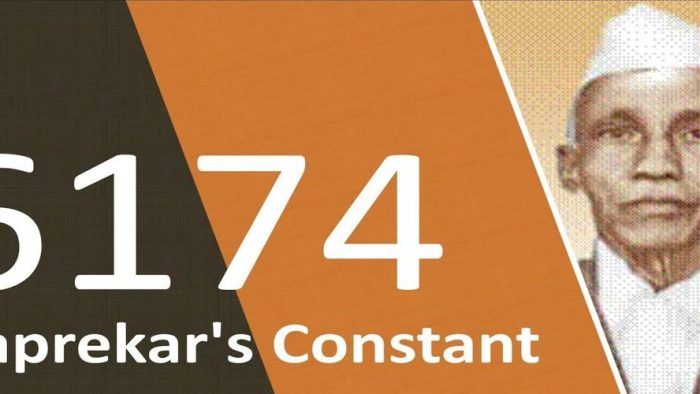# A Primer On Kaprekar’s ConstantI was born in the year 1971. Let us play with this number.

By arranging the digits of this number in descending and ascending order we get two numbers 9711 and 1179. Now let us subtract the number we get by arranging the digits in ascending order from the number we get by arranging the digits in descending order.
1) 9711 – 1179 = 8532
Now let us arrange the digits of the result, 8532, in descending and ascending order. This way we get two numbers 8532 and 2358. Let us subtract 2358 from 8532.
2) 8532 – 2358 = 6174
Let us repeat the same procedure with this new result, 6174.
3) 7641 – 1467 = 6174
Lo behold. We get the same result.

Let us do the same operations on the number of the current year, 2019.
1) 9210 – 0129 = 9081
2) 9810 – 0189 = 9621
3) 9621 – 1269 = 8352
4) 8532 – 2358 = 6174
Again we get the same result, 6174.

Now, let us do the same operations on the number of the year our country gained independence, 1947.
1) 9741 – 1479 = 8262
2) 8622 – 2268 = 6354
3) 6543 – 3456 = 3087
4) 8730 – 0378 = 8352
5) 8532 – 2358 = 6174
Lo behold, again we get the same number, 6174.

If you perform these operations on any four-digit number in which all the digits are not the same (a four-digit number consisting at least two different digits), then you will get the result as 6174 in not more than seven steps. This amazing discovery was made by Indian mathematician Shri Dattatreya Ramchandra Kaprekar (1905 – 1986) in the year 1949. In honour of Shri Kaprekar the number 6174 is called Kaprekar’s constant and the above procedure is called Kaprekar’s operations.

Kaprekar was a mathematics teacher in a school at Deolali (near Nasik) from 1930 to 1962. Number theory (study of properties of integers) was his favourite subject. Due to his passion for mathematics he was also called Ganitanand (one who derives happiness by doing mathematics).

Kaprekar had made many amazing discoveries in number theory. But his work was initially ignored by Indian mathematicians. In 1975, Martin Gardner, a famous author on popular mathematics, wrote an article on Kaprekar’s constant in his popular column on recreational mathematics in Scientific American. This made Kaprekar and his work world famous.

Lastly as a tribute to this great mathematician let us perform Kaprekar’s operations on a new four-digit number, 3133.
1) 3331 – 1333 = 1998
2) 9981 – 1899 = 8082
3) 8820 – 0288 = 8532
4) 8532 – 2358 = 6174

Disclaimer: The opinions expressed in this article belong to the author. Indic Today is neither responsible nor liable for the accuracy, completeness, suitability, or validity of any information in the article.